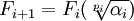## Saturday, October 25, 2008

### Ruffini's Proof: Field Extensions

In today's blog, I will present Paolo Ruffini's proof that if n ≥ 5 and F is a splitting field, then F/k is not a radical tower. This constitutes step two of the Abel-Ruffini proof using field extensions. For a review of splitting fields and field extensions, start here.

Below is Pierre Lauren Wantzel's version of Ruffini's proof. Niels Abel independently presented his own version of this version which I covered previously.

Today's content is taken from Jean-Pierre Tignol's Galois' Theory of Algebraic Equations.

Lemma 1:

Let u,a be functions of n parameters in a field F such that up = a for some prime p.

Let n ≥ 5

Let σ be the following permutation:

x1 → x2 → x3 → x1 and xi → xi for i greater than 3.

so that:

σf(x1, x2, x3, ..., xn) = f(x2, x3, x1, ..., xn)

Let τ be the following permutation:

x3 → x4 → x5 → x3 and xi → xi for i=1,2 and i greater than 5

so that:

τf(x1, x2, x3, x4, x5, ..., xn) = f(x1, x2, x4, x5, x3, ..., xn)

Then:

If a is invariant under the permutations σ and τ, then so is u.

Proof:

(1) From the given, we have up = a where u,a are functions of n parameters.

(2) Applying the permutation σ to both sides gives us:

σ(u)p = σ(a)

(3) Since a is invariant with regard to σ, we have:

σ(u)p = σ(a)= a = up

(4) Dividing both sides by up gives us:

[σ(u)/u]p = 1

(5) Taking the p-th root of each side and multiplying by u gives us:

σ(u) = ζσu

where ζσ is some p-th root of unity.

(6) Applying σ to both sides gives us:

σ2(u) = ζσ2u

(7) Applying σ again we get:

σ3(u) = ζσ3u

(8) Now, we also know from its definition that σ3(u) = u since:

σ3f(x1, x2, x3, x4, ..., xn) = f(x1,x2, x3, x4, ..., xn)

(9) So, that ζσ3 = 1

(10) We can make the same line of argument with τ to show that:

τ(u) = ζτu

where ζτ is a pth root of unity and

ζτ3 = 1

(11) Putting these two results together gives us:

σ*τ(u) = σ(τ(u)) = ζσζτu

σ2*τ = σ2(τ(u)) = ζσ2ζτu

(12) Now, representing each of these as permutations we get:

σ*τ:

x1 → x2 → x3 → x4 → x5 → x1 and xi → xi for i greater than 5.

σ2*τ:

x1 → x3 → x4 → x5 → x2 → x1 and xi → xi for i greater than 5

(13) Using the above permutation maps, we get that:

(σ*τ)5(u) = u

2τ)5(u) = u

(14) Using step #5 and step #10 above, we can use step #13 to conclude that:

σζτ)5 = (ζσ2ζτ)5 = 1

(15) Now, we also note that:

ζσ = ζσ6σ-5 = ( ζσ6σ-5)*(ζτ5τ-5)*(ζσ5σ-5) = ζσ6*(ζτ5σ5)*(ζσ-5σ-5τ-5) =

= ζσ6*(ζσζτ)5σ2ζτ)-5

(16) But then using step #14 with step #15, we have:

ζσ = ζσ6*(ζσζτ)5[(ζσ2ζτ)5]-1 = ζσ6*(1)*(1)-1 = ζσ6

(17) Using step #9 above, we then have:

ζσ =σ3)2 = (1)2 = 1

(18) Now, since σζτ)5 =1, we have:

(1*ζτ)5 =1

so that:

ζτ5 = 1

(19) We can now show that ζτ = 1 since:
ζτ = ζτ6τ-5 = (ζτ3)2*(ζτ5)-1

Taking ζτ3 = 1 (step #10) and ζτ5 = 1 (step #18), we get:

ζτ = τ3)2*(ζτ5)-1 = (1)2*(1)-1 = 1

QED

Theorem 2: Ruffini's Theorem

Let P(X) = (X - x1)*...*(X - xn) = Xn - s1Xn-1 + ... + (-1)nsn = 0

where si are coefficients in field k.

Let K be a field such that K = k(s1, ..., sn)

If n ≥ 5 and F is a splitting field for P(X), then F/K is not a radical tower.

Proof:

(1) Since F is a splitting field for P(X), x1 ∈ F.

(2) Since K is defined around the coefficients of P, it is clear that all elements of K are invariant under the permutations of σ and τ. [See Lemma 2, here]

(3) Assume F/K is a tower of radicals such that:

K = F0 ⊂ F1 ⊂ ... ⊂ Fm-1 ⊂ Fm = F

such that for each 0 ≤ i ≤ m:where pi is a prime and αi ∈ F0 = K

(4) Then, by induction, all elements Fi are also invariant against the permutations σ and τ since:

(a) We know that all elements of F0 = K are invariant under σ and τ (step #2 above) so we can assume that this is true up to some i where i ≥ 0.

(b) Let a = αi, u = a(1/p) so that:

Fi+1 = Fi(u)

and

a ∈ Fi

(c) But since a ∈ Fi, it follows that a is invariant under σ and τ.

(d) Using Lemma 1 above, we note that this implies that u is also invariant under σ and τ.

(e) But then all elements of Fi(u) = Fi+1 are also invariant under σ and τ.

(5) But now we have a contradiction since x1 ∈ F = Fm is not invariant under σ [since σ(x1) = x2]

(6) So, we reject our assumption in step #3.

QED

References
• Michael I. Rosen, "Niels Hendrik Abel and Equations of the Fifth Degree", The American Mathematical Monthly, Vol. 102, No. 6 (Jun. - Jul., 1995), pp 495-595.
• Jean-Pierre Tignol, Galois' Theory of Algebraic Equations, World Scientific, 2001
• Jorg Bewersdorff, Galois Theory for Beginners, American Mathematical Society, 2006.